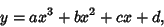## Cubical ParabolaAn equation of the formwhere the three Roots of the equation coincide (and are therefore real), i.e.,See also Cubical Conic Section, Cubical Ellipse, Cubical Hyperbola, Cubical Parabolic Hyperbola, Parabola, Semicubical Parabola